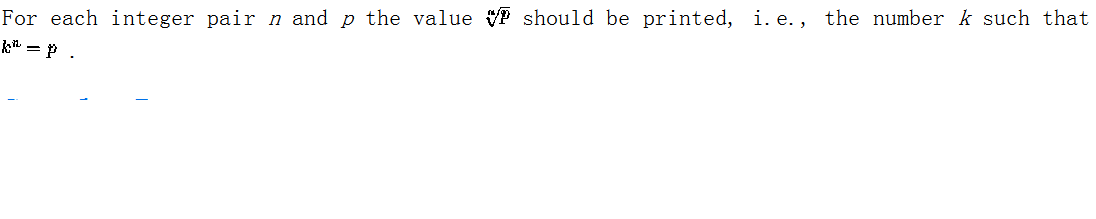# 1513. Power of Cryptography

Background

Current work in cryptography involves (among other things) large prime numbers and computing powers of numbers modulo functions of these primes. Work in this area has resulted in the practical use of results from number theory and other branches of mathematics once considered to be of only theoretical interest.

This problem involves the efficient computation of integer roots of numbers.

The Problem### 输入格式

The input consists of a sequence of integer pairs n and p with each integer on a line by itself. For all such pairs 1<=n<=200 , 1<=p<=10^101 and there exists an integer k, 1<=k<=10^9 such that k^n=p.

### 输出格式### 样例

Input
2
16
3
27
7
4357186184021382204544

Output
4
3
1234


10 人解决，14 人已尝试。

10 份提交通过，共有 16 份提交。

5.4 EMB 奖励。